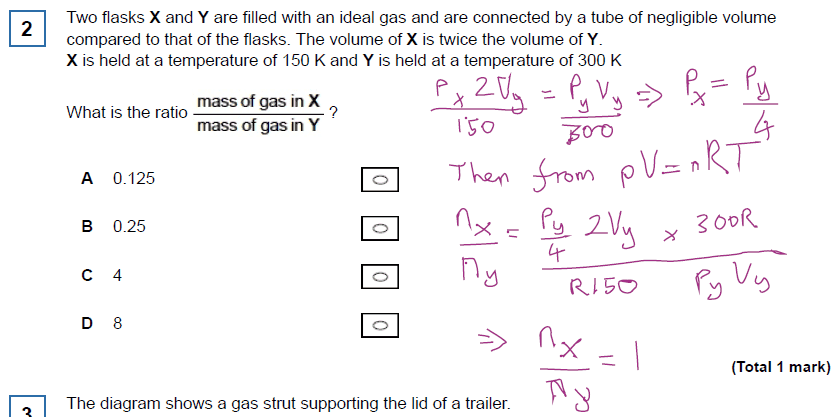# Pressure of an ideal gas -- A Level Multiple Choice Problem

maxelcat
Homework Statement:
Bit stuck on this... AQA question. I know I am doing something wrong but I can't see it

Two flasks X and Y are filled with an ideal gas and are connected by a tube of negligible volume compared to that of the flasks. The volume of X is twice the volume of Y.

X is held at a temperature of 150 K and Y is held at a temperature of 300 K

What is the ratio of mass in x / mass in y

Thanks
Relevant Equations:
(pV/ T)= constant and pV=n RTmaxelcat
Sorry meant to say answer is c

As I understand your work, you start by asserting: $$\frac{P_x2V_y}{150}=\frac{P_yV_y}{300}$$Where does this assertion come from?
You then use the truth of that assertion to conclude that$$P_x=\frac{P_y}{4}$$But the fact that there is a tube connecting the two chambers indicates that in fact, ##P_x=P_y##.
•